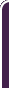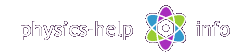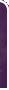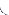homephysics online guidephysics help my info terms of use contactsWhat is Physics? Mechanics Electricity and Magnetism Electric Field Gauss' Law about below subjects Electric Potential Capacity Direct Current Magnetic Field Magnetic Field Laws Magnetic Interactions Electromagnetic Induction Maxwell's Equations Oscillations and Waves Simple Harmonic Motion Damped Harmonic Motion Driven Harmonic Motion Electric Oscillation Alternating Current Wave Motion Elastic Waves Electromagnetic Waves Optics Light Waves Geometrical Optics Interference Polarization Diffraction Fraunhofer Diffraction Dispersion, Absorption, Diffusion Doppler Effect Thermodynamics Ideal Gas Molecular Statistics Transport Phenomena First Law of Thermodynamics Second and Third Laws of Thermodynamics Imperfect Gas Liquids Solids Quantum Physics Thermal Radiation Quantum Properties of Light Wave Properties of Particles Planetary Model of Atom X-Rays Particle in Potential Well Pauli Exclusion Principle Nuclear Physics Solid State Physics Appendices# What is Physics?

SI units & Physics constants

The main task of Physics is to investigate general properties and motion of matter. The term "motion of matter" means any change in position or internal state of matter.

In physics, the matter is everything that can be measured with instruments.  Matter is subdivided into two categories, substance and field.Substance is everything that has rest mass being fixed. So substance is the substantial characteristic of matter

Field is matter with zero rest mass, which exists only in motion. Any interaction inside the substance is produced by means of field. So field is the power characteristic of matter.

The substance is made up of over one hundred known elements, called atoms, combined into the periodic table of chemical elements, from Hydrogen,, to Meitnerium. Atom is the smallest piece of substance that retains its chemical properties.

The atoms consist of elementary particles, such as protons, neutrons, electrons, photons, mesons, muons, neutrino, and etc. There are over 400 different elementary particles known at presence. This number has been increasing year by year

The elementary particle consists of leptons and quarks subdivided into three groups of two leptons and two quarks in each group. This is where the substance breaking down stops at presence. The lepton-quark model is of interest of modern physics.

The tiny central part of any atom, where all its mass is concentrated, is called nucleus. The nucleus is made up of protons and neutrons, which are held tightly in place by strong nucleus force. The nucleus is surrounded by light particles called electrons, which are tied to the nucleus by electric force. In normal state, the number of electrons in any atom is equal to the number of protons. The dimension of nucleus is only about 1/100,000 of the dimension of the atom. If the nucleus were the size of a grain of sand, the atom would be the size of a football field.

The number of protons in the nucleus of atom will dictate the type of the element. Neutron has mass nearly equal to the mass of proton, therefore the number of neutrons defines different isotopes of given element which have the same chemical properties, but differ physically.

The elementary particles interact by means of fields, called as fundamental interactions. These interactions constitute physical basis of any motion of matter in all known phenomena. Investigation of these interactions is of the main interest of modern physics.

All famous interactions of matter are subdivided into four fundamental interactions with main characteristics shown in the below table.

 Interaction Source Relative value Radius of action, r Gravitational Masses 1 Far-ranging (0 < r <) Weak All elementary particles 1023 Short-ranging (r < 10-18 m) Electromagnetic Electric charges 1036 Far-ranging (0 < r <) Strong Heavy particles (hadrons) 1039 Short-ranging (r < 10-15 m)

Physics deals with numerous physical quantities which characterize different properties of objects and can be measured experimentally.

All physical quantities are subdivided into three groups: scalars, vectors, and tensors.

Scalar is a quantity completely specified by one number, called magnitude of the quantity, and appropriate units. Scalar examples: mass, speed, density, temperature, charge, etc.

Vector is a quantity specified by magnitude and appropriate units plus direction in space. Vector is defined by three scalar numbers called components (or coordinates) of the vector. Vector examples: velocity, acceleration, force, momentum, electric field, magnetic field, and etc.

Tensor is a more complex quantity with vectors as components. Tensor examples: strain, stress, moment of inertia, elasticity, polarizability, etc.

The main method in physics is the experiment based on measuring the physical quantities. To explain the results of these experiments the hypotheses are formulated. These hypotheses then are verified and defined more exactly in the next experiments. As a result these hypotheses take shape of physics laws.Physics laws establish objective stable quantitative relations between different physical quantities.

In physics all physical quantities related by unified system of units. The main system of units used in physics nowadays is International System of Units, abbreviated SI and known as the metric system. This system is based on seven base units: meter (m), kilogram (kg), second (s), ampere (A), kelvin (K), mole (mol), candela (cd), and two auxiliary units: radian (rad) and steradian (sr). These units are defined and reproduced by special physical devices called etalons. All other units are derived units. They are defined from the base and auxiliary units by means of physics laws.

The motion of matter can be exhibited in different forms, such as: mechanical, electromagnetic, oscillatory, thermal, quantum, and etc. In accordance with each of these forms of motion, Physics is subdivided into set of independent sections, which are: Mechanics, Electricity and Magnetism, Oscillations and Waves, Optics, Thermodynamics, Quantum Physics.home  |  physics online guide  |  physics help  |  my info  |  terms of use  |  contacts Dr. Nikitin © 2010